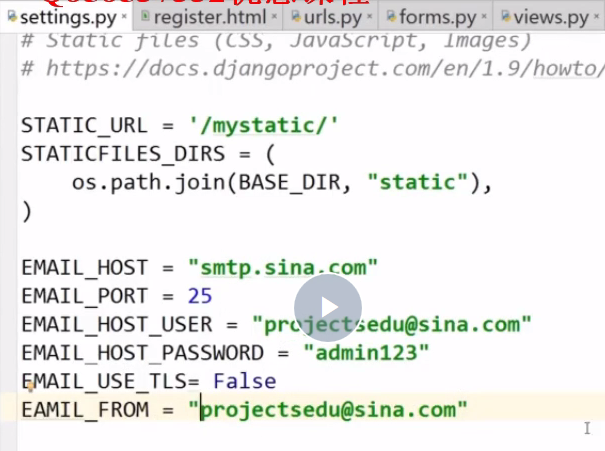Home >  > Django笔记一

## Django笔记一

0

Django简介

Django是一个基于MVC构造的框架。但是在Django中，控制器接受用户输入的部分由框架自行处理，所以 Django 里更关注的是模型（Model）、模板(Template)和视图（Views），称为 MTV模式。它们各自的职责如下：

 层次 职责 模型（Model），即数据存取层 处理与数据相关的所有事务： 如何存取、如何验证有效性、包含哪些行为以及数据之间的关系等。 模板(Template)，即业务逻辑层 处理与表现相关的决定： 如何在页面或其他类型文档中进行显示。 视图（View），即表现层 存取模型及调取恰当模板的相关逻辑。模型与模板的桥梁。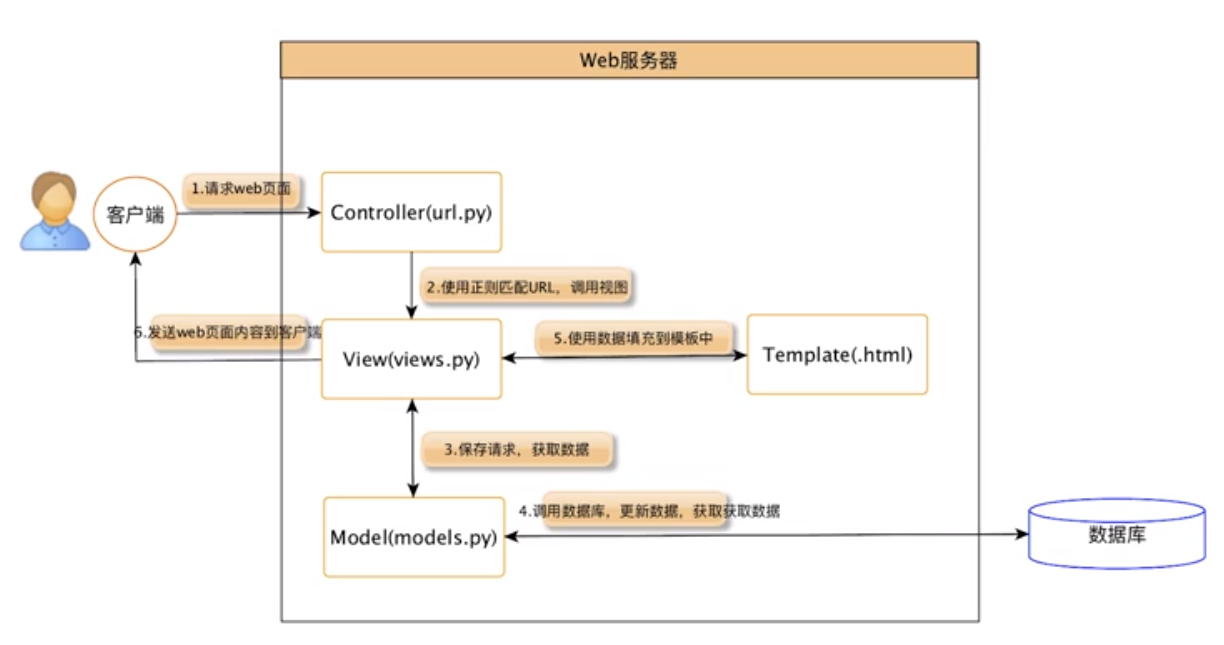http://blog.51cto.com/12814931/2123622
Django框架全面讲解
https://www.cnblogs.com/LiCheng-/p/6920900.html

https://github.com/APSL/puput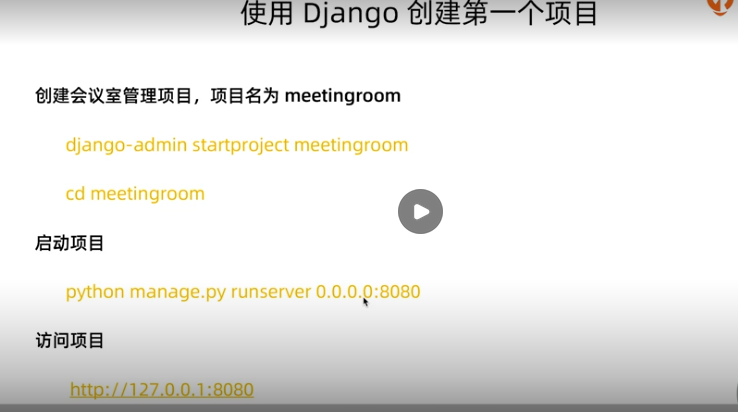1.固定选项

```category = models.CharField(default="pxjg",verbose_name="培训机构",max_lenght=20, choices=(("pxjg","培训机构"),(“pxgr”,"培训个人"),("pxxx","培训学校")))
```

2、charField一定要设定max_lenght，textField不需要输入长度。

3、邮箱、URL
email = models.EmailField(max_length=50,verbose_name="邮箱")
url = models.URLField(max_length=200,verbose_name="访问地址")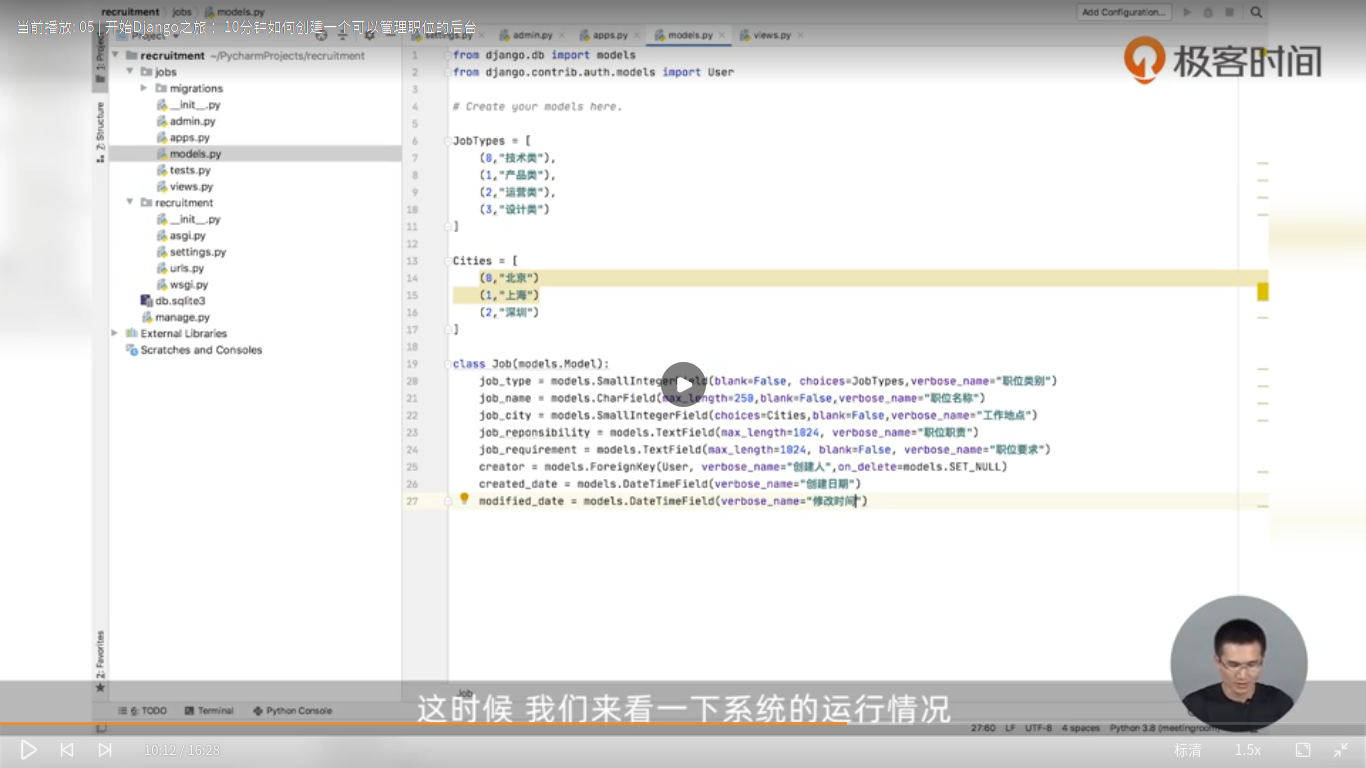3、上传图片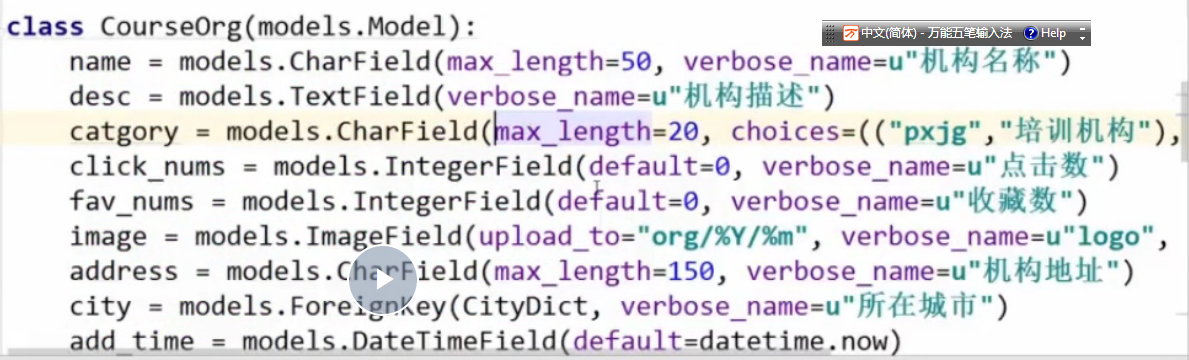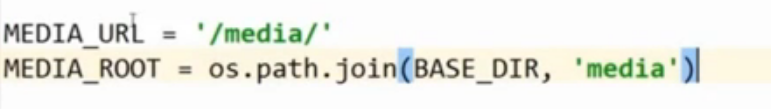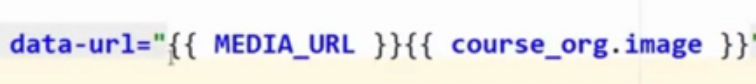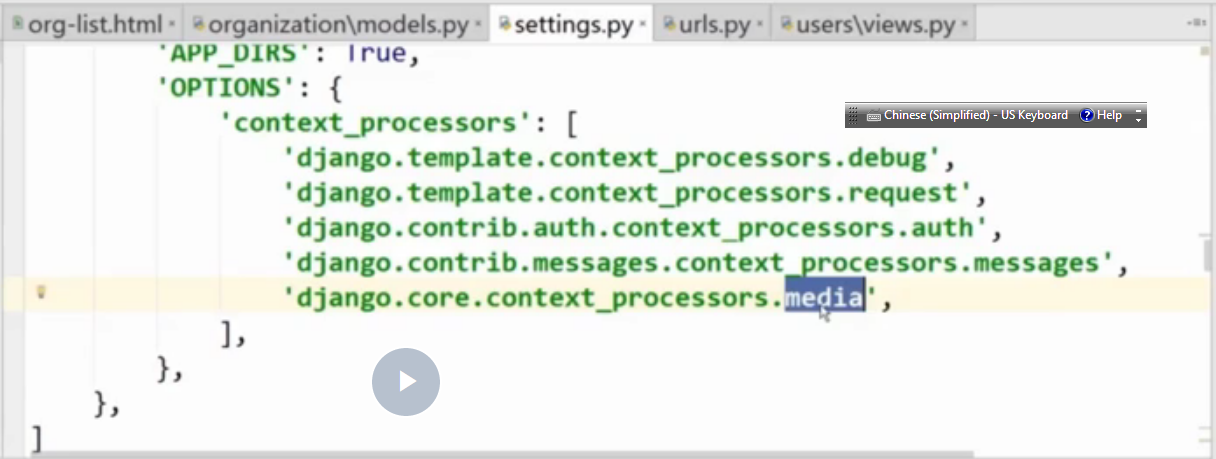```from Mxonline.setting import MEDIA_ROOT
```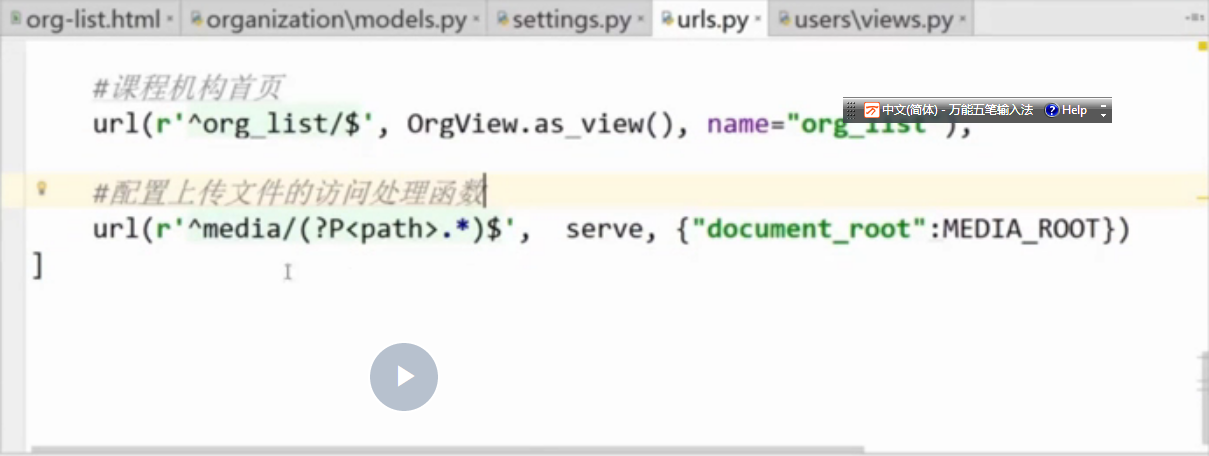4、django的model中，HTMLField字段、DecimailField字段（DecimailField可以明确控制多少位，floatFiedl的位数多了去了）。

null=true 指的是数据库层面
blank = Ture 指的是前端表单提交是否可以为空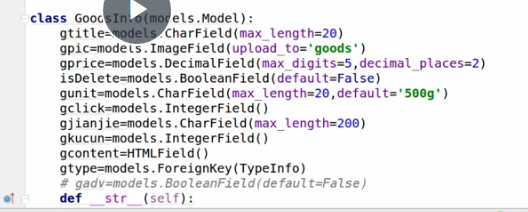5、修改models时，只有更改到数据库，才需要migrate,仅仅影响到python层面的，比如default,blank，不影响数据库结构，不需要migrate。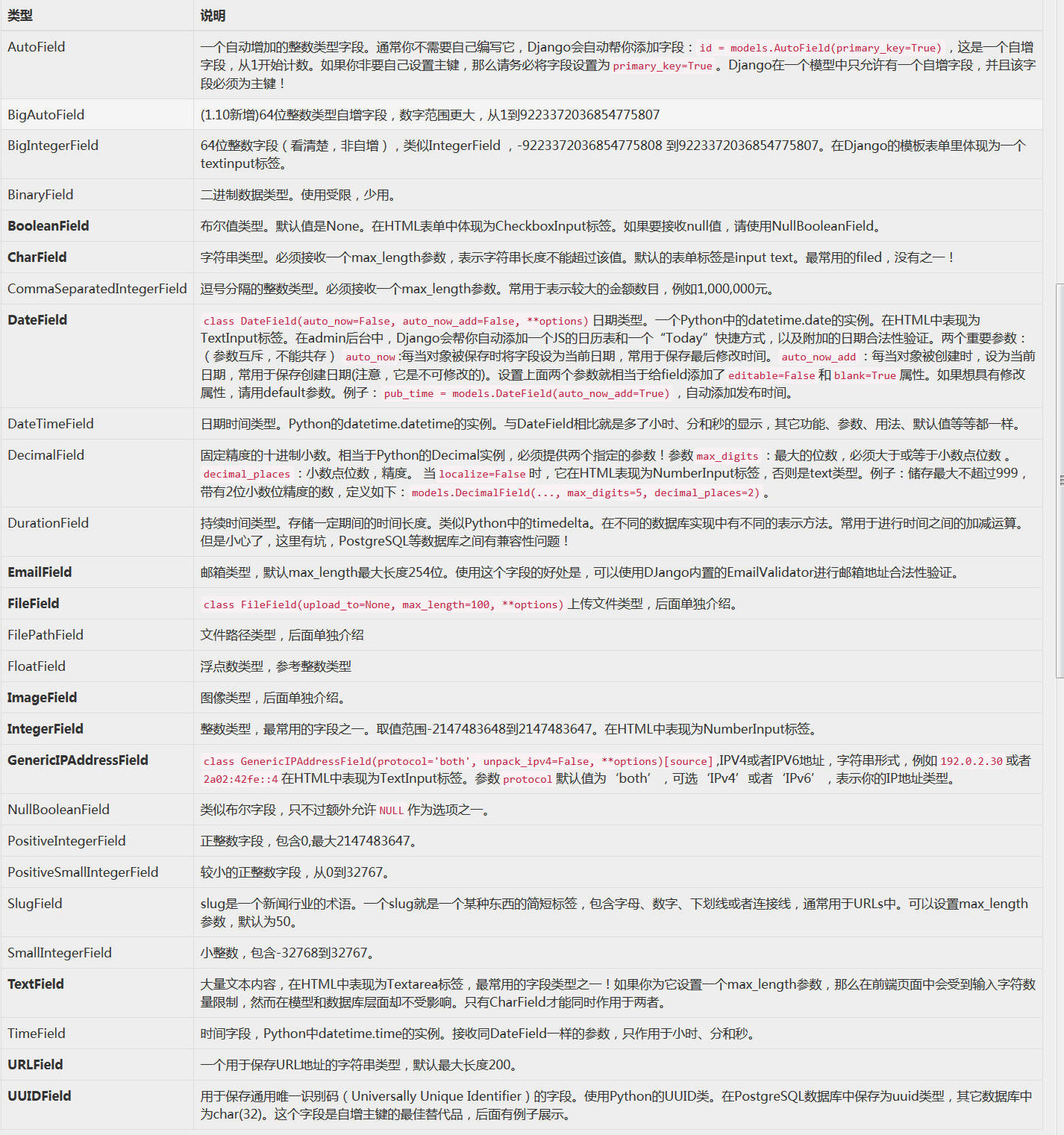6.一些常识

```class Meta:
verbose_name = 'PPT目录管理'
verbose_name_plural = verbose_name
ordering = ("created_at",)
```

```    def __str__(self):
return self.name
```

```from django.db import models
from datetime import datetime
from django.contrib.auth.models import User

from django.utils.translation import gettext_lazy as _

# 候选人学历
DEGREE_TYPE = ((u'本科', u'本科'), (u'硕士', u'硕士'), (u'博士', u'博士'))

JobTypes = [
(0,"技术类"),
(1,"产品类"),
(2,"运营类"),
(3,"设计类"),
(4,"市场营销类")
]

Cities = [
(0,"北京"),
(1,"上海"),
(2,"深圳"),
(3,"杭州"),
(4,"广州")
]

class Job(models.Model):
# Translators: 职位实体的翻译
job_type = models.SmallIntegerField(blank=False, choices=JobTypes, verbose_name=_("职位类别"))
job_name = models.CharField(max_length=250, blank=False, verbose_name=_("职位名称"))
job_city = models.SmallIntegerField(choices=Cities, blank=False, verbose_name=_("工作地点"))
job_responsibility = models.TextField(max_length=1024, verbose_name=_("职位职责"))
job_requirement = models.TextField(max_length=1024, blank=False, verbose_name=_("职位要求"))
creator = models.ForeignKey(User, verbose_name=_("创建人"), null=True, on_delete=models.SET_NULL)
modified_date = models.DateTimeField(verbose_name=_("修改日期"), auto_now=True)

class Meta:
verbose_name = _('职位')
verbose_name_plural = _('职位列表')

def __str__(self):
return self.job_name

class Resume(models.Model):
# Translators: 简历实体的翻译
applicant = models.ForeignKey(User, verbose_name=_("申请人"), null=True, on_delete=models.SET_NULL)
city = models.CharField(max_length=135, verbose_name=_('城市'))
phone = models.CharField(max_length=135,  verbose_name=_('手机号码'))
email = models.EmailField(max_length=135, blank=True, verbose_name=_('邮箱'))
apply_position = models.CharField(max_length=135, blank=True, verbose_name=_('应聘职位'))
gender = models.CharField(max_length=135, blank=True, verbose_name=_('性别'))

# 学校与学历信息
bachelor_school = models.CharField(max_length=135, blank=True, verbose_name=_('本科学校'))
master_school = models.CharField(max_length=135, blank=True, verbose_name=_('研究生学校'))
doctor_school = models.CharField(max_length=135, blank=True, verbose_name=u'博士生学校')
major = models.CharField(max_length=135, blank=True, verbose_name=_('专业'))
degree = models.CharField(max_length=135, choices=DEGREE_TYPE, blank=True, verbose_name=_('学历'))
created_date = models.DateTimeField(verbose_name="创建日期", default=datetime.now)
modified_date = models.DateTimeField(verbose_name="修改日期", auto_now=True)

# 候选人自我介绍，工作经历，项目经历
candidate_introduction = models.TextField(max_length=1024, blank=True, verbose_name=u'自我介绍')
work_experience = models.TextField(max_length=1024, blank=True, verbose_name=u'工作经历')
project_experience = models.TextField(max_length=1024, blank=True, verbose_name=u'项目经历')

class Meta:
verbose_name = _('简历')
verbose_name_plural = _('简历列表')

def __str__(self):
```

related_name用于外键的反向查询，也就是通过父表的一条记录，查询子表的多条记录。

（一）URL的配置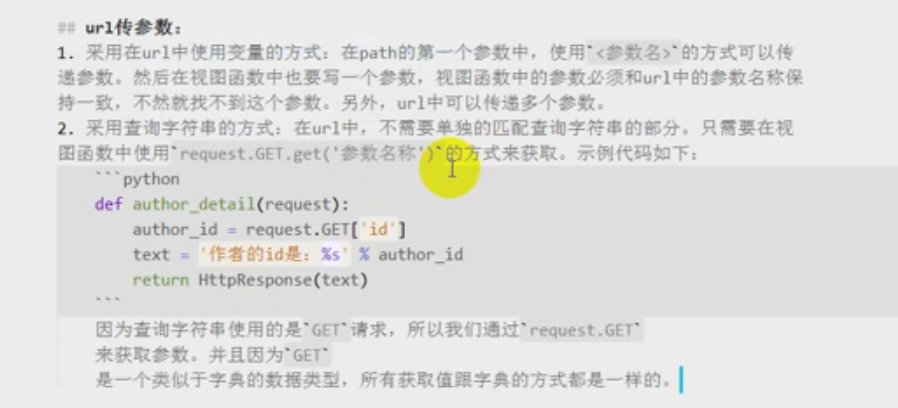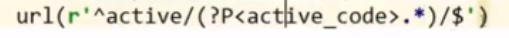（二）利用名称配置url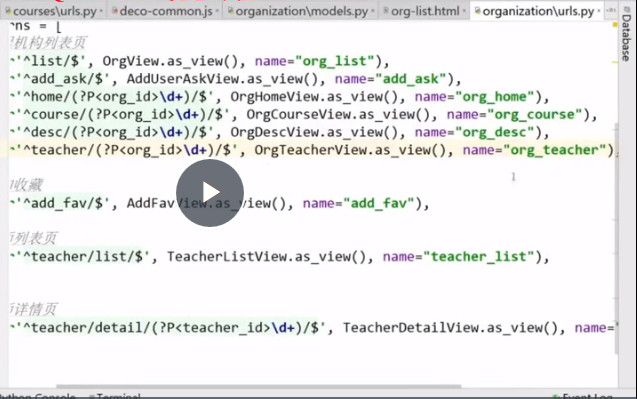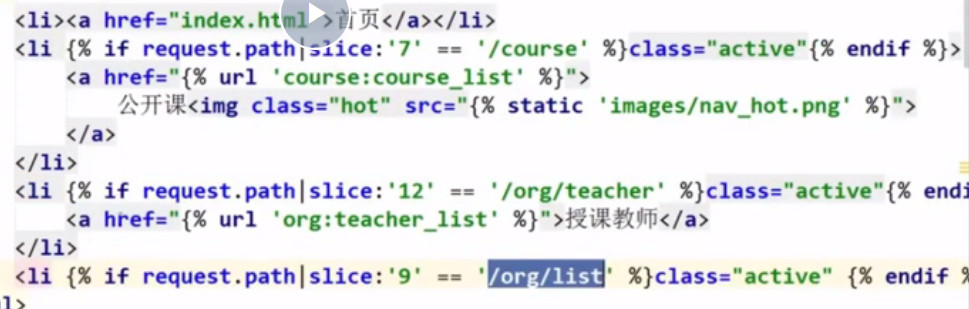```from django.shortcuts import render,HttpResponse

def index(request):
return HttpResponse('hello')
```

```def index(request):
return render(request, 'ppt/index.html')
```

1.基于类的配置方法
(1)例子

```from django.views.generic.base import view
```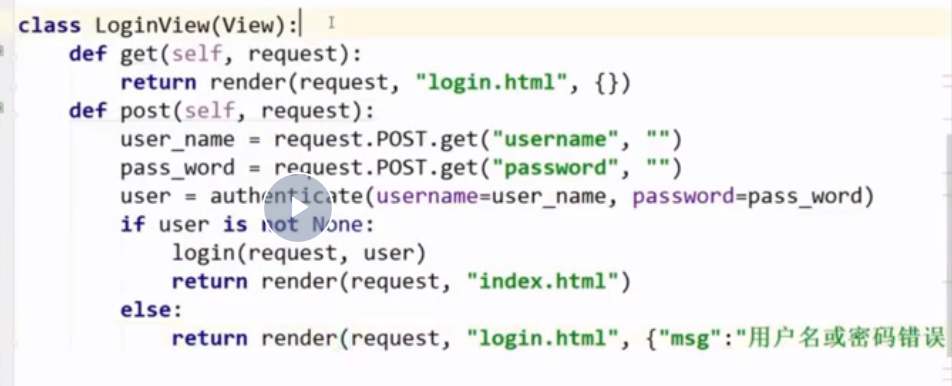（2）相关说明：
A、关键变量。
queryset:用于提取数据，比如可以让提取的数据倒序排列。
context_object_name————在模板中的变量名。比如就是{% for question in questions %}中的questions。
template_name————-模板一般是一个html文件名
paginate_by————如果做分页这个参数说明每页有几个item项
model——————对应的模型（Model）
http_method_names———-请求类型 可以是get或者post

B、方法。

get_queryset——–或者需要展示的数据并且返回（必须要有返回）
get_context_data——–传递额外的数据到模板（html）。

（3）最简单的通用视图：

```from django.views.generic import ListView
from .models import Article

class IndexView(ListView):

model = Article
```

(4)加强版本

```from django.views.generic import ListView
from .models import Article
from django.utils import timezone

class IndexView(ListView):

queryset = Article.objects.all().order_by("-pub_date")
template_name = 'blog/article_list.html'
context_object_name = 'latest_articles'

def get_context_data(self, **kwargs):
context = super().get_context_data(**kwargs)
context['now'] = timezone.now()
return context
```

```from django.views.generic import ListView
from .models import Article
from django.utils import timezone

class IndexView(ListView):

template_name = 'blog/article_list.html'
context_object_name = 'latest_articles'

def get_queryset(self):
return Article.objects.filter(author=self.request.user).order_by('-pub_date')

def get_context_data(self, **kwargs):
context = super().get_context_data(**kwargs)
context['now'] = timezone.now()
return context
```

5.更多的view

2、首页显示多个分类的商品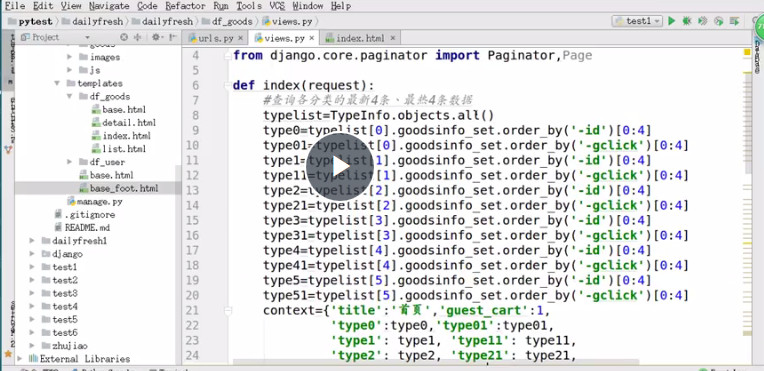3、用get查询，查不到会抛异常，用filter不会。

4.对数据的处理总结：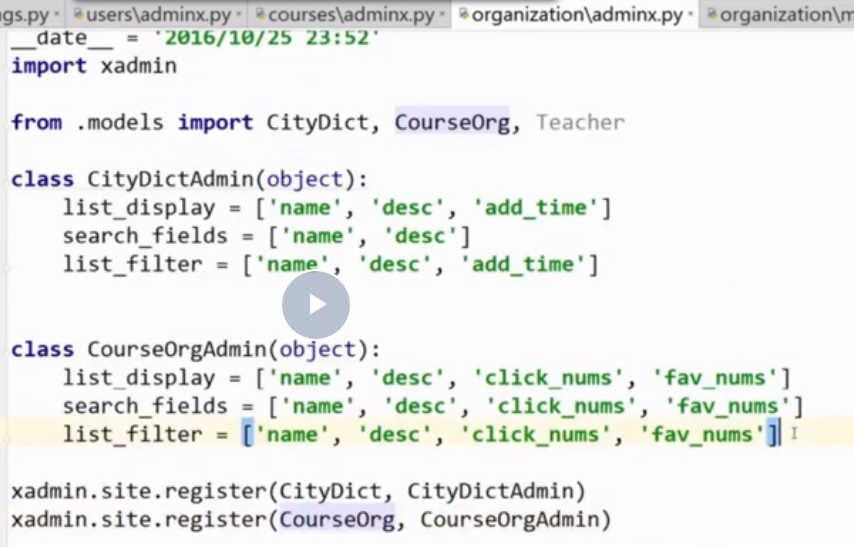F6 单步调试、F8全速前进。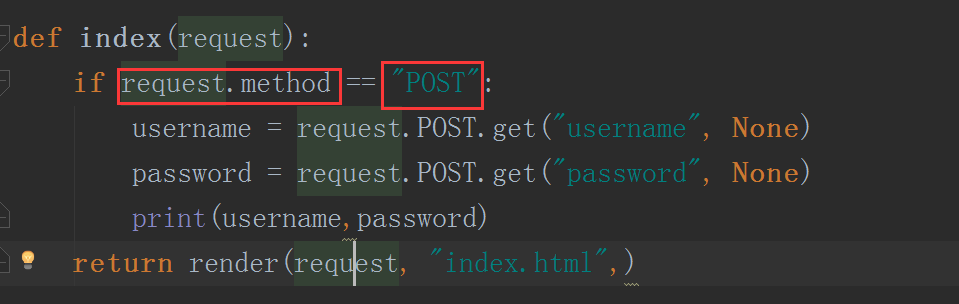```<html lang="en">
<meta charset="UTF-8">
<title>首页</title>
<body>
<h1>用户输入：</h1>
<form action="/index/" method="post">
{% csrf_token %}   <!--加入这行 -->
<input type="submit" value="提交" />
</form>
</body>
</html>
```